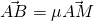Problem Solving Tips #1So these past months, I have been focusing on harnessing students’ abilities to interpret questions properly. Every line in a question is there for a reason and hold little pieces of information. As a student, you need to piece these information together.

Take the following question as an example:

We have a, b and c=a+2b.
Given further that M is on OC, and point A, B, and M are collinear. Find the ratio of OM:OC.

Now this question looks rather short. Many students will first start by drawing to help them see. To be honest, I was tell students that drawing out vectors is not necessary since it doesn’t yield any marks and we spend 10 minutes trying to figure out how it is supposed to look. We are better learning how to read questions.

Let’s start dissecting

M is on OC tells us that.

A, B, and M are collinear tells us that the points are parallel with a common point. NOTE: Collinear is different from parallel. The former is a proper subset of the latter actually.
This tells us thatStudents should have no trouble continuing to solve forand.

So this little exercise is to simply illustrate the importance of learning how to read questions and of course, writing it out.

Not readable? Change text.